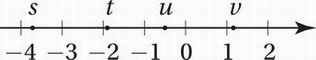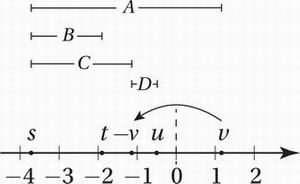# SAT Math Multiple Choice Question 626: Answer and Explanation

### Test Information

Question: 626

11.If s, t, u, and v are the coordinates of the indicated points on the number line above, which of the following is greatest?

• A. |s - v|
• B. |s - t |
• C. |s + v|
• D. |u + v|

Explanation:

A

Algebra (absolute values) MEDIUM-HARD

First, we should notice that each choice can be interpreted as a distance between two points on the number line.

(A) |s - v| = the distance between s and v

(B) |s - t| = the distance between s and t

(C) |s + v| = |s - (-v)| = the distance between s and -v

(D) |u + v| = |u - (-v)| = the distance between u and -v

Thinking this way gives us a very straightforward way to solve the problem without doing any calculation. First we need to locate -v on the number line by just reflecting v over the origin at 0. (Recall that multiplication by -1 is equivalent to reflecting a point on the number line over the origin at 0.) This makes it easy to see the distances the problem is asking us to compare:Clearly, the greatest of these distances is (A).